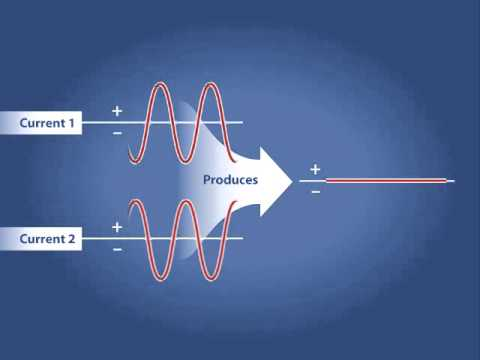### Main Difference

Constructive and Destructive interference are the terms related the waves and the vibrations produced by them. In constructive difference two waves interact and the resulting amplitude is greater than each of the individual wave, whereas in destructive interference when two waves interact the resulting amplitude is smaller than that of the individual waves. Constructive Interference takes place when two waves interact or meet with each other in such a way that displacement of the waves produced remains in the similar direction, whereas destructive interference takes place when the two waves meeting have displacements in the opposite direction. In constructive interference, crests of the two waves meeting each other lines up together, though in destructive interference the crest of one wave interacts with the trough of the other, which results as a lower total amplitude.

### Comparison Chart

 Constructive Interference Destructive Interference Amplitude In constructive difference two waves interact and the resulting amplitude is greater than each of the individual wave. In destructive interference when two waves interact the resulting amplitude is smaller than that of the individual waves. Mechanism Constructive Interference takes place when two waves interact or meet with each other in such a way that displacement of the waves produced remains in the similar direction. Destructive interference takes place when the two waves meeting have displacements in the opposite direction. Crest and trough In constructive interference, crests of the two waves meeting each other lines up together. In destructive interference the crest of one wave interacts with the trough of the other.

### What is Constructive Interference?

In simpler words, constructive interference goes on describing a situation of addition of two waves. As we know that in constructive interference the two waves meet each other and have displacement in the similar direction, this shows that the addition of waves takes place in this concept. In constructive interference, the resulting wave has a higher amplitude than each of the individual wave’s amplitude. The principle of superposition is the principle on which constructive and destructive interference depends upon as in both the interference’s two or more waves travel through the same medium at the same time.

### What is Destructive Interference?

Like the constructive interference, two waves also meet each other in destructive interference. Though in this two waves cancel each other and have displacements in the opposite direction. In destructive interference the crest of one wave interacts with the trough of the other, which results as a lower total amplitude. This can be further elaborated as in destructive interference when two waves interact the resulting amplitude is smaller than that of the individual waves. Noise-canceling headphones are one of the best example of destructive interference as the noisy sound being emitted is cancelled out by the antiphase produced by the headphones.

### Constructive Interference vs. Destructive Interference

• In constructive difference two waves interact and the resulting amplitude is greater than each of the individual wave, whereas in destructive interference when two waves interact the resulting amplitude is smaller than that of the individual waves.
• Constructive Interference takes place when two waves interact or meet with each other in such a way that displacement of the waves produced remains in the similar direction, whereas destructive interference takes place when the two waves meeting have displacements in the opposite direction.
• In constructive interference, crests of the two waves meeting each other lines up together, though in destructive interference the crest of one wave interacts with the trough of the other.